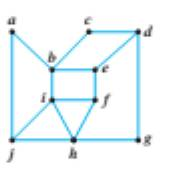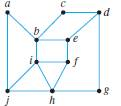Chapter 10.1, Problem 32ES### Discrete Mathematics With Applicat...

5th Edition
EPP + 1 other
ISBN: 9781337694193

#### Solutions

Chapter
Section### Discrete Mathematics With Applicat...

5th Edition
EPP + 1 other
ISBN: 9781337694193
Textbook Problem
22 views

# Show that none of graphs in 31-33 has a Hamiltonian circuit.To determine

To prove:

Show that the graph does not have a Hamiltonian circuit.Explanation

Given information:

Proof:

Given a graph G, from the subgraph H consisting all vertices of degree 2 and their incident edges.

If G has a Hamiltonian circuit H (which we consider as a subgraph of G ), then it must contain H as a subgraph, since a Hamiltonian circuit must include every edge incident on a vertex of degree 2.

If H contains a vertex with degree > 2, then G has no Hamiltonian circuit.

If H has a vertex of degree > 2, then so does H, which is impossible since H is a circuit

### Still sussing out bartleby?

Check out a sample textbook solution.

See a sample solution

#### The Solution to Your Study Problems

Bartleby provides explanations to thousands of textbook problems written by our experts, many with advanced degrees!

Get Started